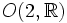# Von Dyck group

This article defines a group property: a property that can be evaluated to true/false for any given group, invariant under isomorphism
View a complete list of group properties
VIEW RELATED: Group property implications | Group property non-implications |Group metaproperty satisfactions | Group metaproperty dissatisfactions | Group property satisfactions | Group property dissatisfactions

This article defines a family of groups whose members are parametrized by tuples of natural numbers. In other words, for every tuple of natural numbers, there is a unique corresponding group (upto isomorphism) in that family

## Definition

### Definition in terms of presentation

For natural numbers$l,m,n$, the von Dyck group$D(l,m,n)$ is defined by the following presentation:$\langle a,b,c|a^l = b^m = c^n = abc = e \rangle$

where$e$ denotes the identity element.

This is a subgroup of index two in the triangle group, but some people use the term triangle group for the von Dyck group.

### Geometric description

Given natural numbers$l,m,n$, consider a triangle with sides$\pi/l, \pi/m, \pi/n$ on a suitable simply connected Riemannian surface (i.e., a suitable model for Euclidean or non-Euclidean geometry). The von Dyck group is the group generated by rotations about the vertices of the triangle by angles of$2\pi/l$,$2\pi/m$,$2\pi/n$ respectively.

## The three types

### Spherical von Dyck groups

The triple$(l,m,n)$ in this case satisfies:$\frac{1}{l} + \frac{1}{m} + \frac{1}{n} > 1$,

The solutions to which are$(2,3,3), (2,3,4), (2,3,5)$, and$(2,2,n)$.

This is the spherical case, with the model being the unit sphere in three-dimensional space, and the corresponding von Dyck groups are termed spherical von Dyck groups. Spherical von Dyck groups are subgroups of the special orthogonal group$SO(3,\R)$, because$SO(3,\R)$ is precisely the group of orientation-preserving isometries of the sphere. All of these turn out to be finite subgroups of$SO(3,\R)$, and these also turn out to be the only finite subgroups of$SO(3,\R)$, a fact that follows from Euler's theorem and some additional work. The finiteness can also be viewed as a consequence of the fact that the sphere is compact and simply connected. Further information: Classification of finite subgroups of SO(3,R)

### Euclidean von Dyck groups

The triple$(l,m,n)$ in this case satisfies:$\frac{1}{l} + \frac{1}{m} + \frac{1}{n} = 1$,

for which the only solutions are$(4,4,2)$ and$(3,3,3)$, i.e., the right isosceles triangle and the equilateral triangle in the usual Euclidean plane.

Both of these give wallpaper groups, and neither is finite.

### Hyperbolic von Dyck groups$\frac{1}{l} + \frac{1}{m} + \frac{1}{n} < 1$,

for which there are infinitely many solutions. The model for this is the hyperbolic plane.

## Particular cases

Smallest parameter Middle parameter Largest parameter Common name for group Group order Symmetry object
1$n$$n$ Cyclic group$n$ Regular polygon, symmetries in$SO(2,\R)$
2 2$n$ Dihedral group$2n$ Regular polygon, symmetries in$O(2,\R)$ or in$SO(3,\R)$
2 3 3 Alternating group:A4$12$ Regular tetrahedron, symmetries in$SO(3,\R)$
2 3 4 Symmetric group:S4$24$ Cube or octahedron, symmetries in$SO(3,\R)$
2 3 5 Alternating group:A5$60$ Icosahedron or dodecahedron, symmetries in$SO(3,\R)$